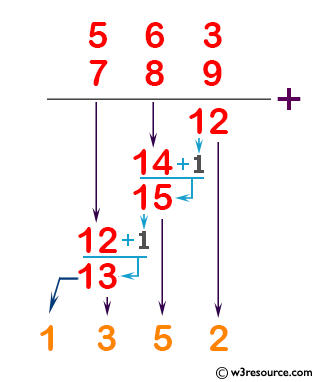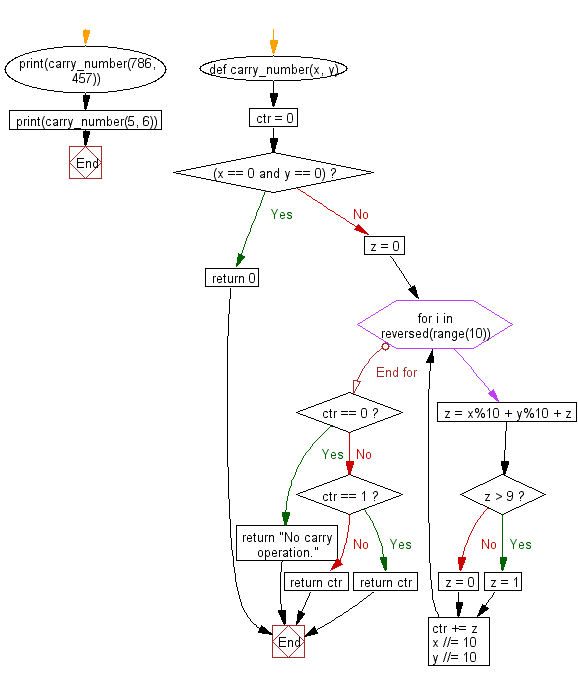﻿ Python: Count the number of carry operations for each of a set of addition problems - w3resource# Python: Count the number of carry operations for each of a set of addition problems

## Python Basic - 1: Exercise-31 with Solution

Write a Python program to count the number of carry operations for each of a set of addition problems.

According to Wikipedia " In elementary arithmetic, a carry is a digit that is transferred from one column of digits to another column of more significant digits. It is part of the standard algorithm to add numbers together by starting with the rightmost digits and working to the left. For example, when 6 and 7 are added to make 13, the "3" is written to the same column and the "1" is carried to the left".

Pictorial Presentation:Sample Solution:

Python Code:

``````def carry_number(x, y):
ctr = 0
if(x == 0 and y == 0):
return 0
z = 0
for i in reversed(range(10)):
z = x%10 + y%10 + z
if z > 9:
z = 1
else:
z = 0
ctr += z
x //= 10
y //= 10

if ctr == 0:
return "No carry operation."
elif ctr == 1:
return ctr
else:
return ctr
print(carry_number(786, 457))
print(carry_number(5, 6))
``````

Sample Output:

```3
1
```

Flowchart:## Visualize Python code execution:

The following tool visualize what the computer is doing step-by-step as it executes the said program:

Python Code Editor:

Have another way to solve this solution? Contribute your code (and comments) through Disqus.

What is the difficulty level of this exercise?

Test your Programming skills with w3resource's quiz.

﻿

## Python: Tips of the Day

Iterating over dictionaries using 'for' loops:

I am a bit puzzled by the following code: d = {'x': 1, 'y': 2, 'z': 3} for key in d: print key, 'corresponds to', d[key] What I don't understand is the key portion. How does Python recognize ...

key is just a variable name.

```for key in d:
```

For Python 3.x:

```for key, value in d.items():
```

For Python 2.x:

```for key, value in d.iteritems():
```

To test for yourself, change the word key to poop.

In Python 3.x, iteritems() was replaced with simply items(), which returns a set-like view backed by the dict, like iteritems() but even better. This is also available in 2.7 as viewitems().

The operation items() will work for both 2 and 3, but in 2 it will return a list of the dictionary's (key, value) pairs, which will not reflect changes to the dict that happen after the items() call. If you want the 2.x behavior in 3.x, you can call list(d.items()).

Ref: https://bit.ly/37dm0Qo